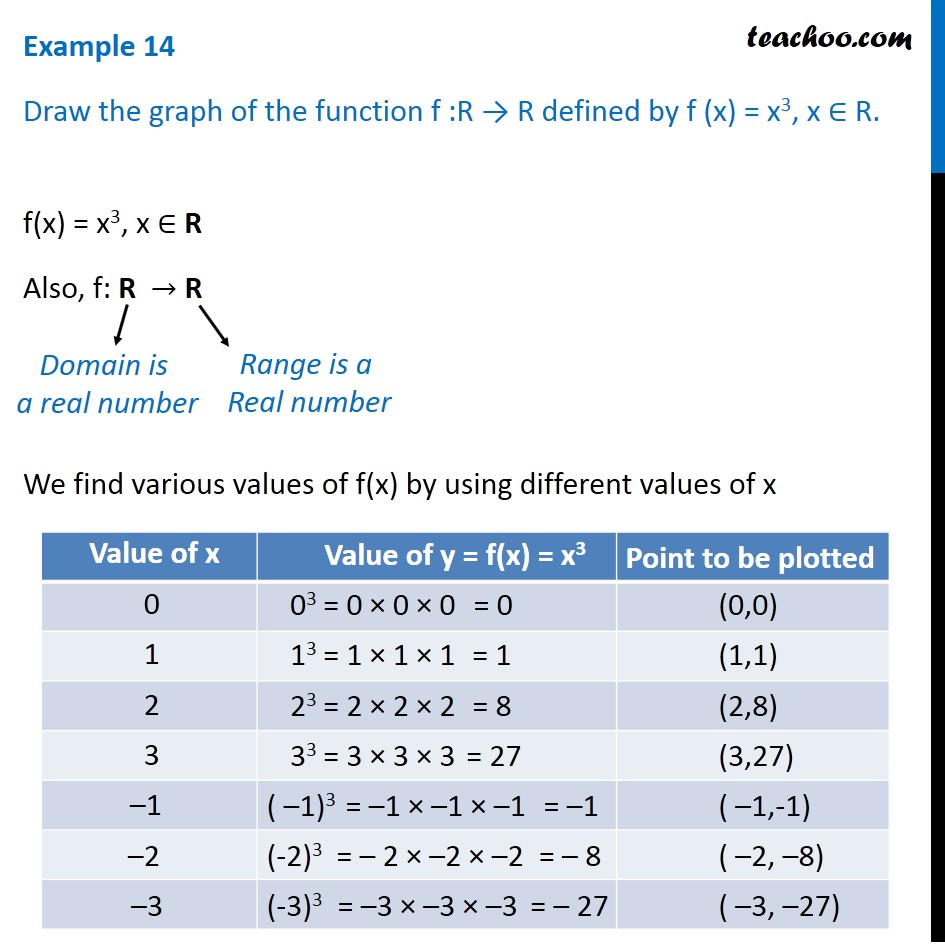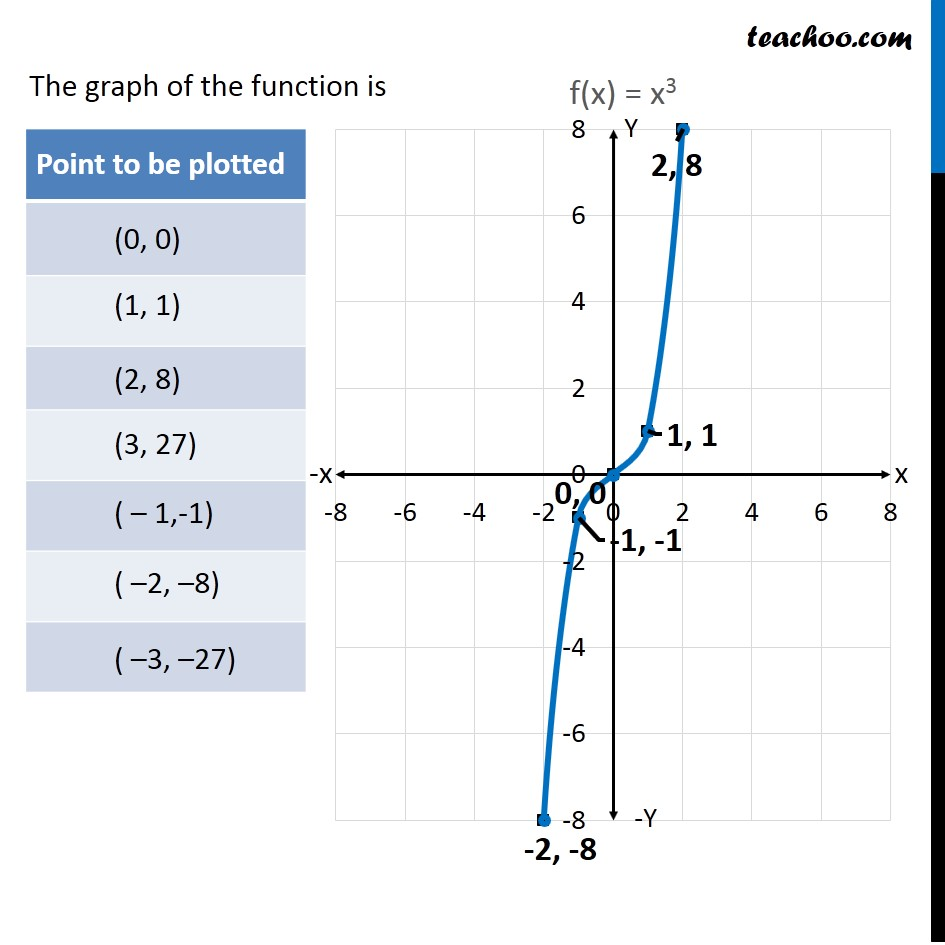Examples

Chapter 2 Class 11 Relations and Functions
Serial order wiseLearn in your speed, with individual attention - Teachoo Maths 1-on-1 Class

### Transcript

Example 14 Draw the graph of the function f :R → R defined by f (x) = x3, x ∈ R. f(x) = x3, x ∈ R Also, f: R → R We find various values of f(x) by using different values of x Domain is a real number Range is a Real number The graph of the function is